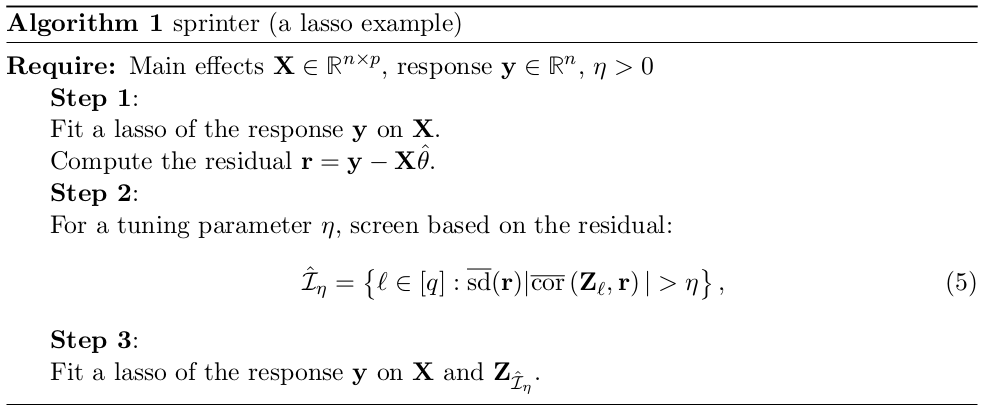# Reluctant Interaction Modeling

##### Posted on Jul 23, 2019
Tags: Variable Selection

Motivated by the large-scale problem sizes, the paper adopt a very simple guiding principle:

One should prefer a main effect over an interaction if all else is equal.

Consider the following two-way interaction model:

$\begin{equation} Y=X^T\beta^* + Z^T\gamma^* + \varepsilon\,,\label{eq:1} \end{equation}$

where $X\in\bbR^p$ is a $p$-dimensional random vector of main effects, $Z=(X_1X_1, X_1X_2,\ldots,X_pX_p)\in\bbR^{(p^2+p)/2}$ is the random vector of all pairwise interactions of $X$.

All pairs lasso (APL) becomes infeasible to compute as $p$ gets large, which takes $O(np^2)$ space.

The paper introduce a computationally viable approach to interaction modeling, called sprinter (for sparse reluctant interaction modeling). not found package yet

• one should prefer main effects over interactions given similar prediction performance. The authors emphasize that this principle is distinct from (although reminiscent of) the common heredity principle really? Sprinter is a multiple-stage method: the first stage it tries to capture as much of the variability in the response as possible without resorting to interactions; in the second stage it includes only interactions that capture signal that cannot be captured by main effects. MARS?
• faster than APL
• finite-sample theoretical properties of sprinter
• hierarchy assumption: an interaction effect is in the model only if either (or both) of the main effects corresponding to the interaction are in the model
• interaction pursuit (IP): first seek a subset of the original $p$ variables that are involved in the nonzero interactions and then restricting attention to interactions between these selected variables.
• easiest when the interactions are concentrated among a small set of original variables, but it would be challenged when there is no such concentration of interactions over a small set of original variables.
• other screening-based methods:
• select interactions based on the partial correlation between the response and each interaction
• screens interactions based on the three-way joint cumulant between the response and two main effects that consists of an interaction.

## A reluctance principle

Consider a simple model

$Y = X_1+X_2+X_1\times X_2\,,$

where $X_1 = 1_A$ and $X_2 = 1_B$. Suppose with high probability $A\subseteq B$, so that $X_1X_2=1_A1_B=1_{A\cap B}\approx 1_A$.

It claims that although the proposed principle and the well-known hierarchical assumption simplify the search of interactions by focusing on certain main effects, the principle does not explicitly tie an interaction to its corresponding main effects concerns

In model \eqref{eq:1}, if $X$ and $Z$ were (so impossible?) uncorrelated, this would be a unique decomposition. However, there can be “overlap” between these two signal terms.

Let $X^T\vartheta^*$ be the part of $Z^T\gamma^*$ that be explained by a linear combination of $X$, i.e.,

$\vartheta^* :=\arg\min_{\vartheta\in\bbR^p} \Var(Z^T \gamma^* -X^T\vartheta) = \Cov(X)^{-1}\Cov(X,Z) \gamma^* = \Sigma^{-1}\Phi \gamma^*\,,$

thus \eqref{eq:1} can be written as

$Y = X^T(\beta^* + \vartheta^* )+W^T \gamma^* + \varepsilon\,,$

where $W=Z-\Phi^T\Sigma^{-1}X$ is the “pure” interaction effects that cannot be captured by linear combinations of $X$.Published in categories Note